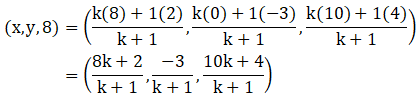A point C with z-coordinate 8 lies on the line segment joining the points A(2, -3, 4) and B(8, 0, 10). Find the coordinates.

Asked by Sakshi | 1 year ago |  36

##### Solution :-

Given:

The points A (2, -3, 4) and B (8, 0, 10)

Let Point C(x, y, 8), and C divides AB in ratio k: 1

So, m = k and n = 1

A(2, -3, 4) and B(8, 0, 10)

Coordinates of C are:On comparing we get,

$$\dfrac{ [10k + 4] }{ [k + 1]}$$ = 8

10k + 4 = 8(k + 1)

10k + 4 = 8k + 8

10k – 8k = 8 – 4

2k = 4

k = $$\dfrac{4}{2}$$

= 2

Here C divides AB in ratio 2:1

x =$$\dfrac{ [8k + 2] }{ [k + 1]}$$

=$$\dfrac{ [8(2) + 2] }{ [2 + 1]}$$

=$$\dfrac{[16 + 2] }{ }$$

$$\dfrac{18}{3}$$

= 6

y = $$\dfrac{-3 }{ [k + 1]}$$

=$$\dfrac{-3 }{ [2 + 1]}$$

$$\dfrac{-3}{3}$$

= -1

The Coordinates of C are (6, -1, 8).

Answered by Aaryan | 1 year ago

### Related Questions

#### A(1, 2, 3), B(0, 4, 1), C(-1, -1, -3) are the vertices of a triangle ABC. Find the point in which the bisector of the

A(1, 2, 3), B(0, 4, 1), C(-1, -1, -3) are the vertices of a triangle ABC. Find the point in which the bisector of the angle ∠BAC meets BC.

#### The mid-points of the sides of a triangle ABC are given by (-2, 3, 5), (4, -1, 7) and (6, 5, 3). Find the coordinates

The mid-points of the sides of a triangle ABC are given by (-2, 3, 5), (4, -1, 7) and (6, 5, 3). Find the coordinates of A, B and C.

#### If the points A(3, 2, -4), B(9, 8, -10) and C(5, 4, -6) are collinear, find the ratio in which C divided AB.

If the points A(3, 2, -4), B(9, 8, -10) and C(5, 4, -6) are collinear, find the ratio in which C divided AB.